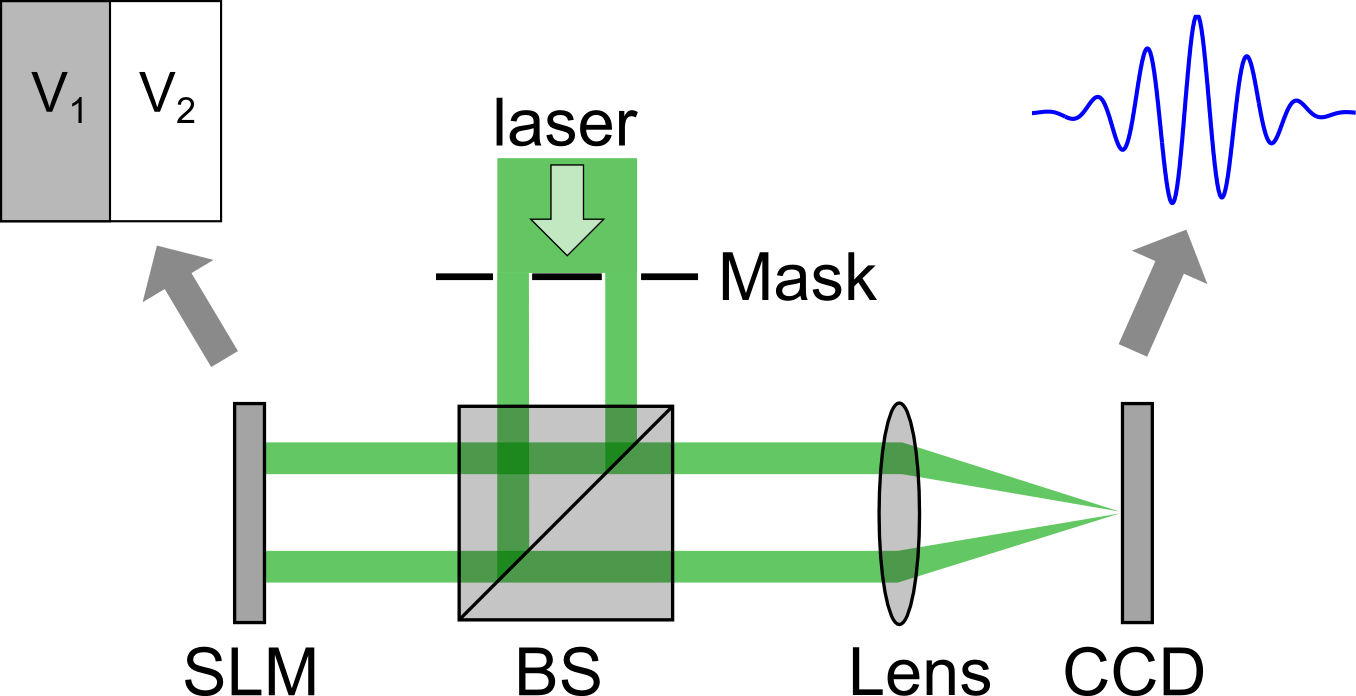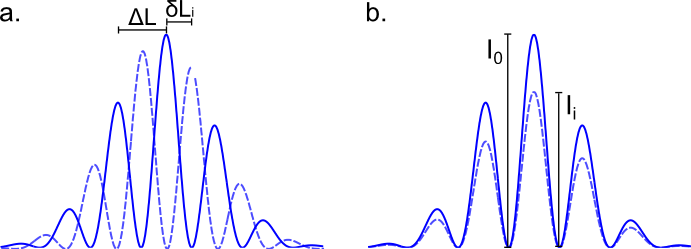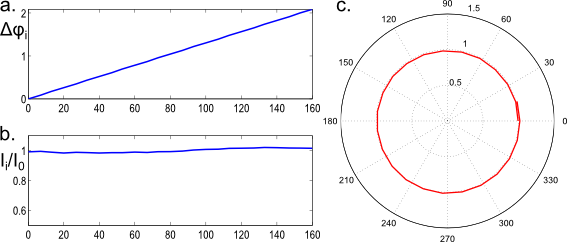## How to characterize and calibrate a phase-only SLM

For most applications in complex media, spatial light modulators are used for their ability to control the phase of a laser beam. Whereas deformable mirrors are insensitive to the input polarization, liquid crystal based SLMs need to work with a given input polarization or sometimes a precise combination of input and output polarizations. It is then necessary for LC SLMs to carefully characterize the modulation to find the setup conditions where amplitude variations are minimal and for which the phase range is at least 2π. In any case, for a given wavelength, it is necessary to know the relation between the value given to a pixel on the SLM and the relative phase shift associated. I present here a typical way to characterize the complex modulation of an SLM.

The principle of the setup is presented in figure 1. A laser beam is expanded and a double hole or double-slit mask is used to have two separated and small collimated beams. The SLM is divided into two parts where the pixel values are respectively V1 and V2. We make sure that the two beams are reflected off the two different parts of the SLM. After modulation by the SLM, we place a digital camera at the focal plane of a lens to observe the interference fringes. It is necessary to have fringes large enough so that there are as many pixels as possible in the inter-fringe distance. We then proceed in two steps for the characterization of the phase and the intensity modulation.Figure 1. Schematic of a typical setup to characterize the complex modulation of a SLM

To measure the phase modulation, we fix the value of V1 - for instance at the minimum pixel value - and vary the value V2 = Vi between the minimal and the maximal pixel value. When the relative phase between the two beams is modified, the fringes maximums will be shifted by a distance δLi (figure 2.a.). δLi  can be obtained by calculating the correlation function between the first and the ith image of the fringes and finding its maximum. The phase variation is given by:

$$\Delta \phi_i=2\pi\frac{\Delta L}{\delta L_i}$$

For a fine measure of the phase modulation, the inter-fringe distance needs to be large enough compare to the pixel size of the camera. For a number N of pixels in ΔL, the minimal phase shift one can measure equals to 2π/N.Figure 2. (a.) Measure of the phase modulation. (b.) Measure of the intensity modulation.

To measure the intensity modulation, we take V1 = V2 = Vi and span again the whole range of pixel values. The intensity variation of the fringes directly gives the intensity modulation (figure 2.b.)

We show in figure 3.a. and b. typical phase and intensity modulations measured for the right input polarization with a Hamamatsu X10468-01. Figure 3.b shows the modulation on a polar representation. A phase-only modulation corresponds to staying on the unitary circle.Figure 3. Typical phase (a.) and intensity (b.) modulation of a phase-only SLM. (c.) Polar representation of the modulation.

It is to be noted that we assumed that the phase introduced by the SLM is nuniform when all the pixels are set to the same value. It is usually not the case for LC-based SLMs and a grayscale image is provided by the manufacturer that compensates the imperfections of the device. The fluctuations of the phase due to this effect are usually on the scale of a large number of pixels. In the previous setup, if the lateral size of the two beams is too large, this effect can not be disregarded and should be taken into account.

Created by sebastien.popoff on 04/05/2013